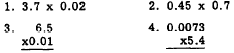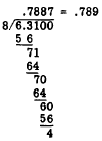Custom SearchMultiplication of Two Decimals To show the rule for multiplying two decimals together, we multiply the decimal in fractional form first and then in the conventional way, as in the following example: 0.4 x 0.37 Writing these decimals as common fractions, we haveIn decimal form the problem isThe placing of the decimal point is reasonable, since 4 tenths of.37 hundredths is a little less than half of 37 hundredths, or about 15 hundredths. Consider the following example: 4.316 x 3.4 In the common fraction form, we haveIn the decimal form the problem isWe note that 4 and a fraction times 3 and a fraction yields a product in the neighborhood of 12. Thus, the decimal point is in the logical place. In the above examples it should be noted in each case that when we multiply the decimals together we are multiplying the numerators. When we place the decimal point by adding the number of decimal places in the multiplier and multiplicand, we are in effect multiplying the denominators. When the numbers multiplied together are thought of as the numerators, the decimal points may be temporarily disregarded and the numbers may be considered whole. This justifies the apparent disregard for place value in the multiplication of decimals. We see that the rule for multiplying decimals is only a modification of the rule for multiplying fractions. To multiply numbers in which one or more of the factors contain a decimal, multiply as though the numbers were whole numbers. Mark off as many decimal places in the product as there are decimal places in the factors together. 348.2 x 0.001 = 0.3482 Practice problems. Multiply as indicated:Answers: 1. 0.074  2. 0.315 3. 0.065  4. 0.03942 Multiplying by Powers of 10 Multiplying by a power of 10 (10, 100, 1,000, etc.) is done mechanically by simply moving the decimal point to the right as many places as there are zeros in the multiplier. For example, 0.00687 is multiplied by 1,000 by moving the decimal point three places to the right as follows: 1,000 x 0.00687 = 6.87 Multiplying a number by 0.1, 0.01, 0.001, etc., is done mechanically by simply moving the decimal point to the left as many places as there are decimal places in the multiplier. For example, 348.2 is multiplied by 0.001 by moving the decimal point three places to the left as follows: 348.2 x 0.001 = 0.3482 DIVISION When the dividend is a whole number, we recognize the problem of division as that of converting a common fraction to a decimal. Thus in the example 5 + 8, we recall that the problem could be writtenThis same problem may be worked by the following, more direct method:Since not all decimals generated by division terminate so early as that in the above example, if at all, it should be predetermined as to how many decimal places it is desired to carry the quotient. If it is decided to terminate a quotient at the third decimal place, the division should be carried to the fourth place so that the correct rounding off to the third place may be determined. When the dividend contains a decimal, the same procedure applies as when the dividend is whole. Notice the following examples (rounded to three decimal places): 1. 6.31 82. 0.0288 32Observe in each case (including the case where the dividend is whole), that the quotient contains the same number of decimal places as the number used in the dividend. Notice also that the place values are rigid; that is, tenths in the quotient appear over tenths in the dividend, hundredths over hundredths, etc. Practice problems. In the following division problems, round off each quotient correct to three decimal places. 1. 10 6 2. 23.5 16 3. 2.743 77 4. 1.00 3 Answers: 1. 1.667 2. 1.469 3. 0.036 4. 0.333Integrated Publishing, Inc. - A (SDVOSB) Service Disabled Veteran Owned Small Business